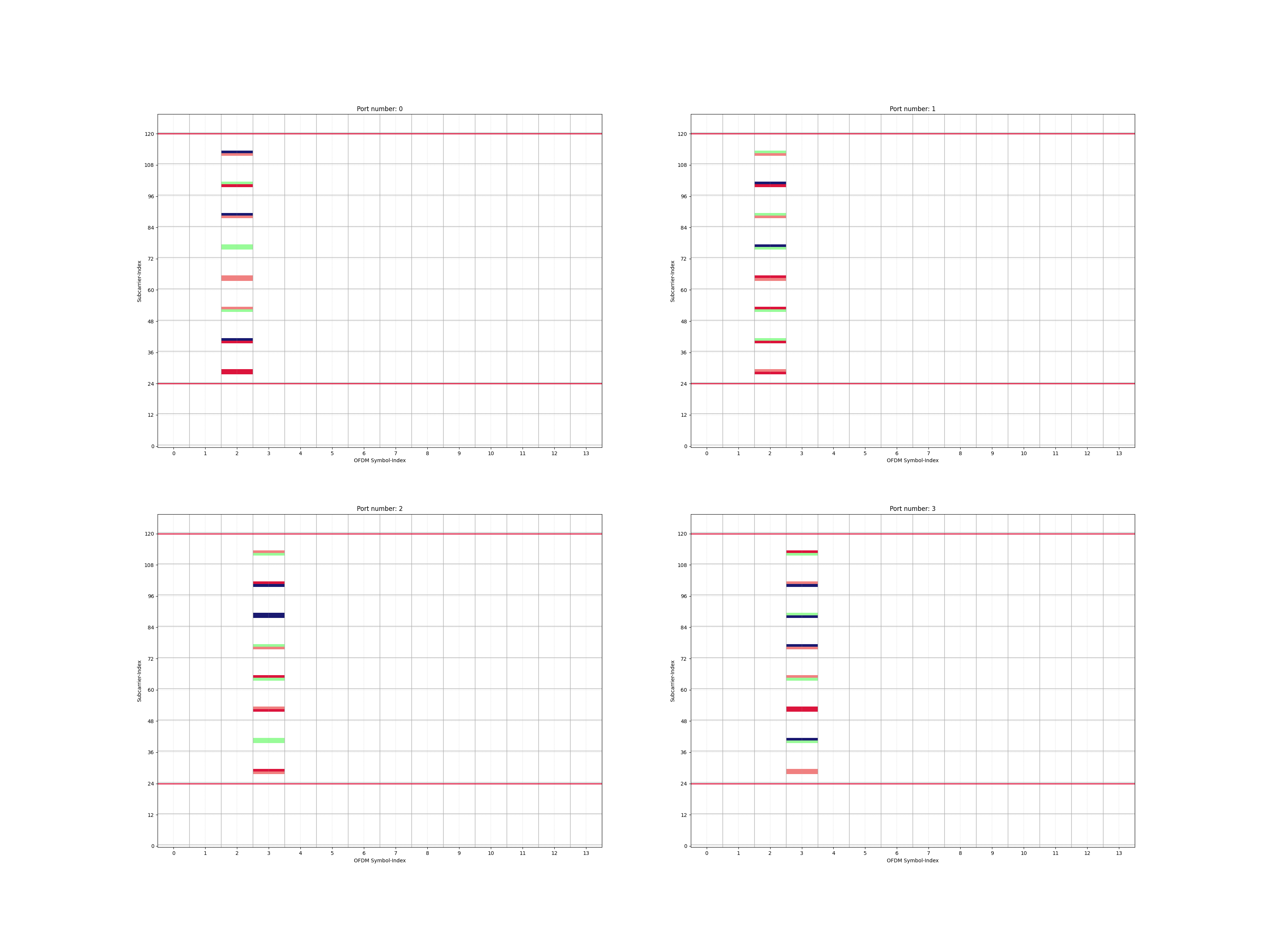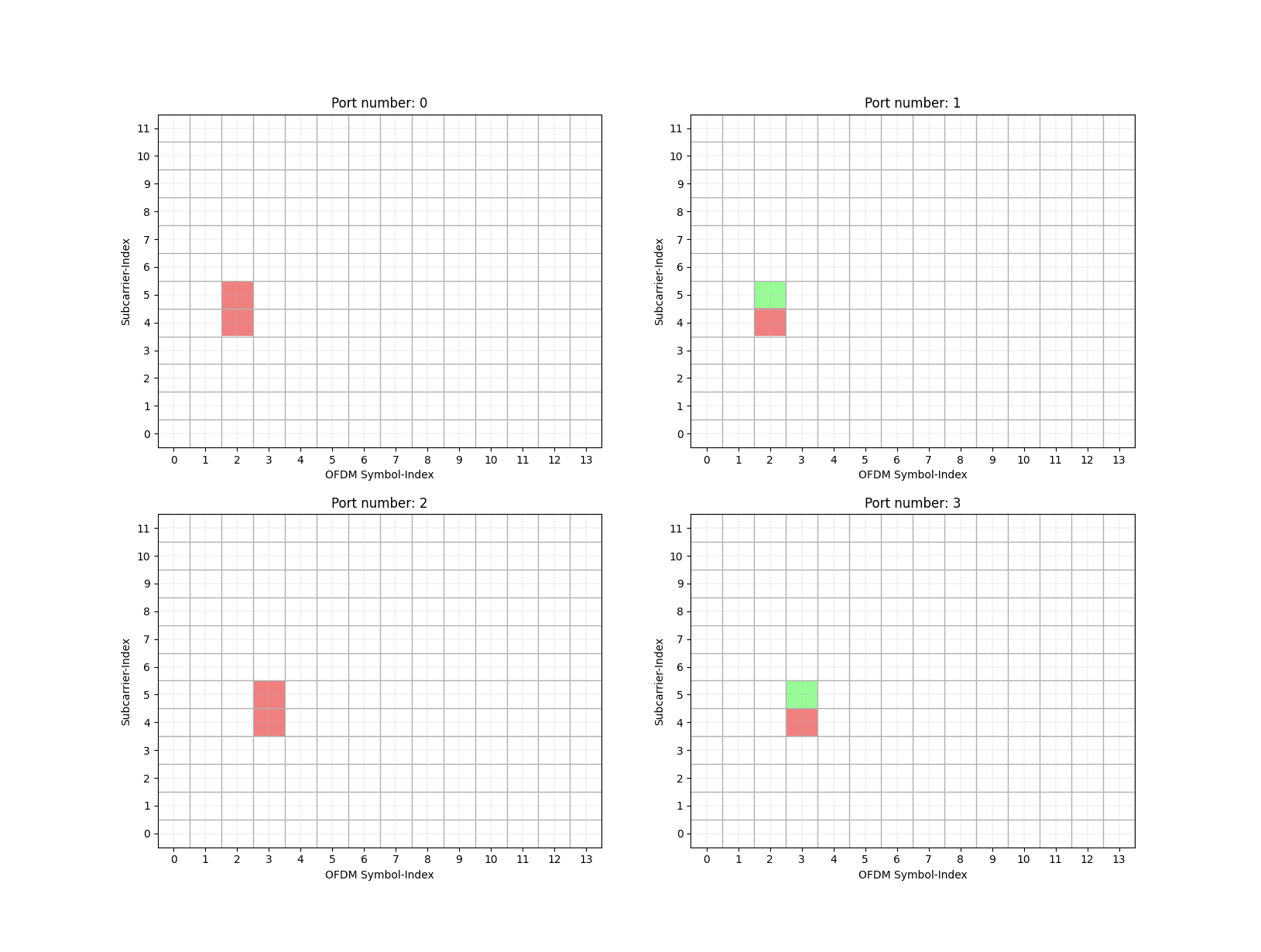# Channel state Information reference signal (CSI-RS)

This module generates the channel state information reference signal (CSI-RS) based on the inputs passed and map this signal to time-frequency (t-f) resource grid as per Section 7.4.1.5 of [3GPPTS38211_csirsRM]. THis resource grid can be orthogonal frequency division multiplexed (OFDM) and transmitted over the wireless channels.

Generating CSI-RS Resource Grid:

subcarrierSpacing = 30000
startPRB          = 2
nrofPRBs          = 8
numSubcarriers    = 12*(nrofPRBs+startPRB)
Nfft              = 128

## CSI-RS Resource Configurations___
rowIndex          = 5
nrofPorts         = 4
density           = 1
cdmType           = "fd-CDM21"

## CSI-RS Parameters__________________________________________
scramblingID      = 13254
numSlotsPerFrame  = int(10*(subcarrierSpacing/15000))
slotNumber        = 5
#_____________________________________________________________

## Time and Frequency resources____________________
betaCSIRS                    = 1
firstOFDMSymbolInTimeDomain  = 2
firstOFDMSymbolInTimeDomain2 = None
frequencyDomainAllocation    = np.array([0,0,1,0,0,0])

## CSI-RS Resource mapping
rmCSIRS   = ResourceMapperCSIRS(nrofPorts, density, cdmType, scramblingID, slotNumber)
csirsGrid = rmCSIRS(betaCSIRS, frequencyDomainAllocation, firstOFDMSymbolInTimeDomain,
firstOFDMSymbolInTimeDomain2, nrofPRBs, startPRB, Nfft)


Display the CSI-RS Resource Grid:

fig0, ax0 = rmCSIRS.displayResourceGrid()Display the CSI-RS CDM Grid:

fig1, ax1 = rmCSIRS.displayCDMPattern()class toolkit5G.ResourceMapping.ResourceMapperCSIRS(nrofPorts, density, cdmType, scramblingID, slotNumber)[source]

Generates the resource grid for CSI-RS as per Section 7.4.3 [3GPPTS38211_SSBGrid].

Parameters:
• nrofPorts (int) – Defines number of ports $$\in \{1,2,4,8,12,16,$$ $$24,32\}$$.

• density (int) – Defines the density of CSI-RS $$\in \{0.5,1,3\}$$.

• cdmType (int) – Defines the CDM Type $$\in \{\text{"noCDM"}, \text{"fd-CDM2"}$$, $$\text{"fd-CDM21"}, \text{"cdm4-FD2-TD2"}, \text{"cdm8-FD2-TD4"}\}$$.

• scramblingID (int) – Defines the scramblingID $$\in \{0,1,\dots,4095\}$$.

• slotNumber (int) – Defines the slot number within a frame $$n_{s,f}^{\mu} \in \{0,1,2,$$ $$\dots,10*2^{\mu}-1\}$$ where $${\mu = \frac{\Delta f}{15 kHz}}$$.

Important

The combination of nrofPorts, density and cdmType shall be selected based on Table 7.4.1.5.3-1 of [3GPPTS38211_csirsRM].

Important

For row 5 and 7 of Table 7.4.1.5.3-1 from [3GPPTS38211_csirsRM] cdmType should be configured as “fd-CDM21”.

Input:
• beta (float) – Defines power scaling factor (default value = 1) of CSI-RS.

• frequencyDomainAllocation ([N,], int) – Defines frequencyDomainAllocation (default value = None) used for selecting $$k_i$$. It is used for determining REs(Resource Elements) for the selected CSI-RS.

• firstOFDMSymbolInTimeDomain (int) – Defines firstOFDMSymbolInTimeDomain (default value = 0) used for selecting $$l_0$$. It is used for determining OFDM symbol Indices for the selected CSI-RS.

• firstOFDMSymbolInTimeDomain2 (int) – Defines firstOFDMSymbolInTimeDomain2 (default value = None) used for selecting $$l_1$$. It is used for determining OFDM symbol Indices for the selected CSI-RS.

• nrofPRBs (int (default value = None)) – Defines number of physical resource blocks (PRBs) occupied by CSI-RS.

• startPRB (int (default value = 0)) – Defines starting physical resource blocks (PRBs) from where next nrofPRBs are occupied by CSI-RS.

• numSubcarrier (int) – Defines frequency size of CSI-RS Resource Grid (default value = None).

Output:

[nrofPorts, 14, numSubcarrier], np.complex64 – Channel state information Reference Signal (CSI-RS) Grid.

Raises:
• ValueError – [Error in ResourceMapperCSIRS]: firstOFDMSymbolInTimeDomain must be an integer from interval [0,13]!

• ValueError – Invalid choice for cdmType!

• Warning – [Warning in ResourceMapperCSIRS]: firstOFDMSymbolInTimeDomain2 is redundant!

• ValueError – [Error in ResourceMapperCSIRS]: firstOFDMSymbolInTimeDomain2 must be an integer from interval [2,12]!

• ValueError – [Error in ResourceMapperCSIRS]: firstOFDMSymbolInTimeDomain2 must be passed for ‘Row’ = ” + str(self.__rowIndex) + “!

• ValueError – [Error in ResourceMapperCSIRS]: For rowIndex = ” + str(self.__rowIndex) + “, length of FrequencyDomainAllocation must be ” + str(numSCmap[self.__rowIndex]) + “!

• ValueError – [Error in ResourceMapperCSIRS]: Elements of frequencyDomainAllocation must be 0 or 1!

• ValueError – [Error in ResourceMapperCSIRS]: frequencyDomainAllocation must be integer or integer array!

• ValueError – [Error in ResourceMapperCSIRS]: rowIndex must take an integer from interval [1,18]!

• ValueError – [Error in ResourceMapperCSIRS]: rowIndex must be integer or integer array!

• ValueError – [Error in ResourceMapperCSIRS]: numSubcarrier must be a positive integer!

• ValueError – [Error in ResourceMapperCSIRS]: numSubcarrier is inconsistent with (startPRB, nrofPRBs)!

• ValueError – [Error in ResourceMapperCSIRS]: numSubcarrier must be a positive integer!

• ValueError – [Error in ResourceMapperCSIRS]: startPRB must be a positive integer!

• ValueError – [Error in ResourceMapperCSIRS]: nrofPRBs must be a positive integer!

• ValueError – [Error in ResourceMapperCSIRS]: For nrofPorts = ” + str(self.__nrofPorts) + ” and density = ” + str(self.__density) + “, cdmType must take value from set: ” + str(possibleCDMtype) + ” !

• ValueError – [Error in ResourceMapperCSIRS]: For nrofPorts = “+str(self.__nrofPorts)+”, density must take value from set: “+str(possibleDensity)+” !

• ValueError – [Error in ResourceMapperCSIRS]: nrofPorts must be interger taking value from set {1,2,4,8,12,16,24,32} !

• ValueError – Error: Number of REs per symbols can’t be more than 2!

• ValueError – Invalid Length of frequencyDomainAllocation!

• ValueError – Invalid number of symbols configured for CSI-RS!

displayCDMPattern(portIndex=None, displayGrid=True)[source]

Provides the method to display CSI-RS CDM grid.

Input:

portIndex (int) – Define the Index of port whose grid has to be displayed. In case no value is passed, grids for all the ports is displayed.

displayResourceGrid(portIndex=None, displayGrid=True)[source]

Provides the method to display CSI-RS resource grid.

Input:

portIndex (int) – Define the Index of port whose grid has to be displayed. In case no value is passed, grids for all the ports is displayed.

References:
[3GPPTS38211_csirsRM] (1,2,3)

(3GPP TS 38.211 version 17.1.0 Release 17) 5G;NR;Physical channels and modulation ‘5.2.2 Low-PAPR sequence generation type 1’